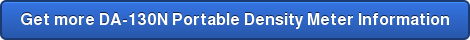DA-130N Portable Density Meter Review

Watch this video to learn more about the very capable DA-130N Portable Density Meter.The DA-130N portable density meter has twelve measurement modes and includes density of alcohol

Hand held density meters are very useful tools.  You can take them with you and perform any of these 12 tasks.

 1) Density 5) API 9) Baume 2) Comp. Density 6) Brix 10) Plato 3) SG(t/t) 7) Alcohol 11) Proof 4) SG 8) H2SO4 12) Conc.

(1) Measurement of density

(2) Measurement of temperature compensated density

(3) Measurement of specific gravityMeasurement of true specific gravity at displayed measurement temperature

(4) Measurement of specific gravity temperature compensatedMeasurement of true specific gravity at preset temperature

(5) Measurement of API degreeMeasurement of density or API degree compensated in temperature to 15 degree C or 60 degree F for the product group A, B or D.

Selection of temperature, 15 degree C or 60 degree F, will be automatically made when setting the temperature unit. Product group A: Crude oil

[Measurement of density: API A (Density) Product group B: Fuel, Petroleum products

[Measurement of density: API B (Density) Product group D: Lubricant

[Measurement of density: API D (Density)

(6) Measurement of Brix concentration

Measurement of API degree: API A (Degree)] Measurement of API degree: API B (Degree)] Measurement of API degree: API D (Degree)]

Measurement of Brix concentration (sucrose concentration expressed in weight %) based on density at 20 degree C(7) Measurement of Alcohol concentration

Measurement of alcohol concentration of Ethanol/Water mixed system in wt % or vol % at 15 degree C or 20 degree C calculated from the density at the measurement temperature. When setting of temperature unit is Fahrenheit

Measurement of alcohol concentration of Ethanol/Water mixed system in wt % or vol % at 60 degree F calculated from the density at the measurement temperature.

(8) Measurement of sulfuric acid concentration

Measurement of sulfuric acid concentration in weight % from density measured at 20 degree C

(9) Measurement of Baume degree

Measurement of Baume degree at converted temperature from density value measured at the measurement temperature

(10) Measurement of Plato degree

Measurement of Plato degree at 20 degree C from density obtained at measurement temperature

(11) Measurement of Proof degree

Measurement of Proof degree at 60 degree F from density obtained at measurement temperature Proof degree is one of the units for alcohol content, and there is US Proof and British Proof

Proof (US) : Measure in US Proof unit (100v/v%=200 US Proof)
Proof (IP) : Measure in UK Proof unit (100v/v%=175 British Proof)

(12) Concentration measurement by setting desired concentration conversion formula